# Conic

Jump to: navigation, search

A second-order curve, i.e. the set of points in a (projective, affine or Euclidean) plane whose homogeneous coordinates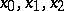(with respect to some projective, affine or Cartesian coordinate system) satisfy an equation of the second degree:The symmetric bilinear formis called the polar form of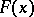. Two pointsand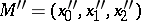for whichare said to be polar conjugates with respect to the conic. If the line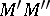intersects the conic at pointsand if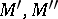are polar conjugates with respect to the conic, thenform a harmonic quadruple. The only self-conjugate points are the points of the conic itself. The pole of a given line with respect to a conic is the point that is polar conjugate with all the points of the line. The set of points in the plane that are polar conjugate with a given point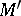with respect to a conic is called the polar ofwith respect to the conic. The polar ofis defined by the linear equation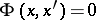in the coordinates. If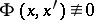, the polar ofis a straight line; if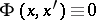, the polar ofis the whole plane. In this caselies on the conic and is called a singular point of the conic. If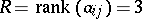, the conic has no singular points and is said to be non-degenerate or to be non-decomposing (non-splitting). In the projective plane this is a real or imaginary oval. A non-degenerate conic defines a correlation on the projective plane, i.e. a bijective mapping from the set of points onto the set of lines. A tangent to a non-degenerate conic is the polar of the point of tangency. If, the conic is a pair of real or imaginary straight lines intersecting at a singular point. If, every point of the conic is singular and the conic itself is a pair of coincident real straight lines (a double line). The affine properties of a conic are distinguished by the specific nature of its location and by the points and lines associated with it with respect to the distinguished line— the line at infinity. A conic is of hyperbolic, elliptic or parabolic type according to whether it intersects the line at infinity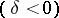, does not intersect itor is tangent to it. Here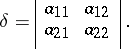The centre of a conic is the pole of the line at infinity, a diameter is the polar of a point at infinity, an asymptote is a tangent to the conic at a point at infinity. Two diameters are conjugate with respect to the conic if their points at infinity are polar conjugates with respect to the conic.

The metric properties of a conic are determined from its affine properties by the invariance of the distance between two arbitrary points. The diameter of a conic that is orthogonal to the conjugate diameter is an axis of symmetry of the conic and is called an axis. A directrix of a conic is the polar of a focus.